4.8.1 Aside: Deletion by Merging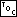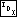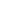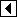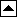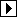The libavl algorithm for deletion is commonly used, but it is also seemingly ad-hoc and arbitrary in its approach. In this section we'll take a look at another algorithm that may seem a little more uniform. Unfortunately, though it is conceptually simpler in some ways, in practice this algorithm is both slower and more difficult to properly implement.

The idea behind this algorithm is to consider deletion as breaking the links between the deleted node and its parent and children. In the most general case, we end up with three disconnected BSTs, one that contains the deleted node's parent and two corresponding to the deleted node's former subtrees. The diagram below shows how this idea works out for the deletion of node 5 from the tree on the left:

Of course, the problem then becomes to reassemble the pieces into a single binary search tree. We can do this by merging the two former subtrees of the deleted node and attaching them as the right child of the parent subtree. As the first step in merging the subtrees, we take the minimum node r in the former right subtree and repeatedly perform a right rotation on its parent, until it is the root of its subtree. The process up to this point looks like this for our example, showing only the subtree containing r: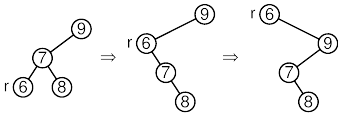Now, because r is the root and the minimum node in its subtree, r has no left child. Also, all the nodes in the opposite subtree are smaller than r. So to merge these subtrees, we simply link the opposite subtree as r's left child and connect r in place of the deleted node: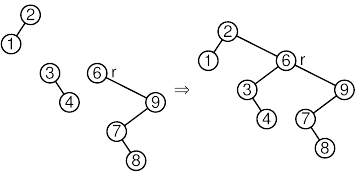The function outline is straightforward:

```45. <BST item deletion function, by merging 45> =
void *bst_delete (struct bst_table *tree, const void *item) {
struct bst_node *p;   /* The node to delete, or a node part way to it. */
struct bst_node *q;	/* Parent of p. */
int cmp, dir;         /* Result of comparison between item and p. */

assert (tree != NULL && item != NULL);

<Step 1: Find BST node to delete by merging 46>
<Step 2: Delete BST node by merging 47>
<Step 3: Finish up after BST deletion by merging 48>

return (void *) item;
}
```

First we search for the node to delete, storing it as p and its parent as q:

```46. <Step 1: Find BST node to delete by merging 46> =
p = (struct bst_node *) &tree->bst_root;
for (cmp = -1; cmp != 0;      cmp = tree->bst_compare (item, p->bst_data, tree->bst_param))   {
dir = cmp > 0;
q = p;
if (p == NULL)
return NULL;
}
```

This code is included in 45.

The actual deletion process is not as simple. We handle specially the case where p has no right child. This is unfortunate for uniformity, but simplifies the rest of the code considerably. The main case starts off with a loop on variable r to build a stack of the nodes in the right subtree of p that will need to be rotated. After the loop, r is the minimum value in p's right subtree. This will be the new root of the merged subtrees after the rotations, so we set r as q's child on the appropriate side and r's left child as p's former left child. After that the only remaining task is the rotations themselves, so we perform them and we're done:

```47. <Step 2: Delete BST node by merging 47> =
if (p->bst_link != NULL)   {
struct bst_node *pa[BST_MAX_HEIGHT]; /* Nodes on stack. */
unsigned char da[BST_MAX_HEIGHT];    /* Directions moved from stack nodes. */
int k = 0;                           /* Stack height. */

struct bst_node *r; /* Iterator; final value is minimum node in subtree. */

for (r = p->bst_link; r->bst_link != NULL; r = r->bst_link)       {
if (k >= BST_MAX_HEIGHT)           {
bst_balance (tree);
return bst_delete (tree, item);
}

pa[k] = r;
da[k++] = 0;
}

for (; k > 0; k–)       {
struct bst_node *y = pa[k - 1];
struct bst_node *x = y->bst_link;
if (k > 1)
pa[k - 2]->bst_link[da[k - 2]] = x;
}
}
```

This code is included in 45.

Finally, there's a bit of obligatory bookkeeping:

```48. <Step 3: Finish up after BST deletion by merging 48> =
item = p->bst_data;
tree->bst_alloc->libavl_free (tree->bst_alloc, p);
tree->bst_count–;
tree->bst_generation++;
```

This code is included in 45.